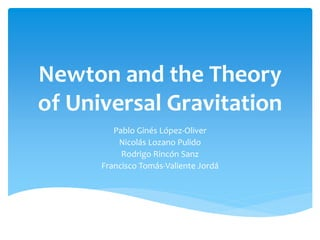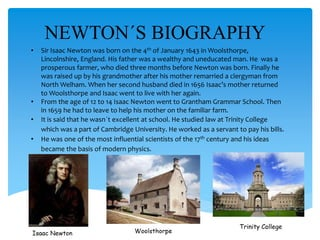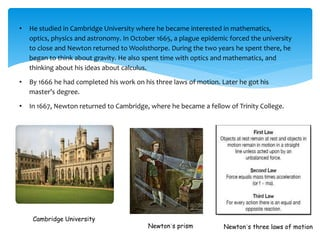Successfully reported this slideshow.

# Newton and his universal theory of gravitation×

# Newton and his universal theory of gravitation

Newton and his universal theory of universal gravitation. This Project explains what this theory is, how Newton proposed it and some other information about Isaac Newton.

Newton and his universal theory of universal gravitation. This Project explains what this theory is, how Newton proposed it and some other information about Isaac Newton.

### Newton and his universal theory of gravitation

1. 1. Newton and the Theory of Universal Gravitation Pablo Ginés López-Oliver Nicolás Lozano Pulido Rodrigo Rincón Sanz Francisco Tomás-Valiente Jordá
2. 2. NEWTON´S BIOGRAPHY • Sir Isaac Newton was born on the 4th of January 1643 in Woolsthorpe, Lincolnshire, England. His father was a wealthy and uneducated man. He was a prosperous farmer, who died three months before Newton was born. Finally he was raised up by his grandmother after his mother remarried a clergyman from North Welham. When her second husband died in 1656 Isaac’s mother returned to Woolsthorpe and Isaac went to live with her again. • From the age of 12 to 14 Isaac Newton went to Grantham Grammar School. Then in 1659 he had to leave to help his mother on the familiar farm. • It is said that he wasn´t excellent at school. He studied law at Trinity College which was a part of Cambridge University. He worked as a servant to pay his bills. • He was one of the most influential scientists of the 17th century and his ideas became the basis of modern physics. Trinity College
3. 3. • He studied in Cambridge University where he became interested in mathematics, optics, physics and astronomy. In October 1665, a plague epidemic forced the university to close and Newton returned to Woolsthorpe. During the two years he spent there, he began to think about gravity. He also spent time with optics and mathematics, and thinking about his ideas about calculus. • By 1666 he had completed his work on his three laws of motion. Later he got his master’s degree. • In 1667, Newton returned to Cambridge, where he became a fellow of Trinity College. Cambridge University Newton´s prism Newton´s three laws of motion
4. 4. • Later on, he was focused on the diffraction of light (he used a prism to discover that white light is made up of the spectrum of colours), universal gravitation, centrifugal force, centripetal force, and on the effects and characteristics of bodies in motion. • He was best known for his work on gravity. He also invented better reflecting lenses for telescopes. Among his greatest “inventions” was calculus. Maths and algebra weren´t enough to explain the ideas in his head, so he invented calculus. • Newton continued studying the notion of gravity and he applied it to the motions of the Earth, sun and moon. • He also made many contributions to optics and calculus, whose founder he has been claimed to. Diffraction of light Newton´s calculus manuscripts Law of universal gravitation
5. 5. • Newton's Principia formulated the laws of motion and universal gravitation. It dominated scientists' view of the physical universe for the next three centuries. Newton removed the last doubts about the theory of the heliocentric model of the cosmos. This theory also demonstrated that the motion of objects on Earth and of celestial bodies could be described by the same principles. • Newton also built the first practical reflecting telescope and , as mentioned before, he developed a theory of colour based on the observation that a prism decomposes white light into the colours of the visible spectrum. He formulated an empirical law of cooling, studied the speed of sound, and introduced the notion of a Newtonian fluid. • In addition to his work on calculus, as a mathematician Newton contributed to the study of power series, generalised the binomial theorem to non-integer exponents, developed Newton's method for approximating the roots of a function, and classified most of the cubic plane curves. Newton's Principia theory of the heliocentric model of the cosmos Newtonian fluid
6. 6.  From the mid-1660s, Newton conducted a series of experiments on the composition of light, and he established the modern study of optics (behaviour of light).  Newton's reflecting telescope, made in 1668, finally brought him to the attention of the scientific community and in 1672 he was made a fellow of the Royal Society. In 1669 Isaac Newton became Lucasian professor of mathematics ( mathematics teacher at Cambridge University) .  In 1687, with the support of his friend the astronomer Edmond Halley, Newton published his greatest work, 'Philosophiae Naturalis Principia Mathematica' ('Mathematical Principles of Natural Philosophy'). This showed how a universal force, gravity, is applied to all objects in all parts of the universe. It set out his theory of gravity.  In 1704, Newton published 'The Opticks' a book about light and colour. He also studied and published works on history, theology and alchemy. Newton's reflecting telescope Royal Society The Opticks Philosophiae Naturalis Principia Mathematica
7. 7. • In 1689, Newton was elected Member of Parliament for Cambridge. In 1696, he was named warden (guard) of the Royal Mint, which was settled in London. He took his duties at the Mint very seriously and he made campaigns against corruption and inefficiency within the organisation. In 1703, he was elected president of the Royal Society, a position he held until his death. Then, he was knighted in 1705. • He was a devout but unorthodox Christian. Beyond his work on the mathematical sciences, Newton dedicated a lot of his time to the study of biblical chronology and alchemy, but most of his work in those areas remained unpublished until long after his death. • Newton was a difficult man, he had many depressions and he was often involved in arguments with other scientists. However, by the early 1700s he was the dominant figure in British and European science. He died on 31 March 1727 at the age of 84 and was buried in Westminster Abbey. Isaac Newton Royal Mint Astronomer Edmond Halley Isaac Newton buried in Westminster Abbey
8. 8. Acceleration of gravity In physics, we refer to gravitational acceleration as the acceleration on an object caused by gravitation. Not taking friction into account (such as air resistance), gravity force (whose acceleration is acceleration of gravity) is the only force applied on an object on free fall on a body big enough to have its own weight. In other words, mass of the object or composition do not interfere in free fall movements. Acceleration of gravity changes slightly in relation with altitude. From 9.78 m/s2 to 9.83 m/s2. But we take as a conventional standard value: 9.80665 m/s2. Practically though, we can observe that objects with low density and big surface fall slower because of air force of friction and such.
9. 9. The force of gravity is the force applied by the gravitational field generally of a massive object on any body within the range of this field. This force is dependent on three factors: the mass of the massive object (usually the Earth or another planet to make it simple), the mass of the smaller body (the object attracted by the planet), and the separation between the two (usually not important in leaser problems because the smaller object is usually within the atmosphere). Actually, this force is established between any two objects because, as Newton stated it is universal. However, when we talk about force of gravity, we usually refer to the force exerted by a massive object, since the force of gravity exerted by an object whose mass is very small is extremely little.
10. 10. In late XVII century Kepler stated that the planets revolved around the Sun following elliptical orbits. However he couldn’t explain why they did, why did they not alter their orbits or why satellites did not crash with their planets. This is when Newton started thinking about it and when the famous story of the apple is supposed to have happened. He didn’t invent gravity, it already existed. But he applied it universally and explained this way why the moon revolved around the Earth and the Earth around the Sun.
11. 11. Newton stated that if you throw a stone with enough force and in the correct angle it will keep revolving forever. This theory explains why the Moon revolves around the Earth without crashing with it.
12. 12. Inverse square law  Newton proposed that gravity was universal.  He proved this by showing that the reason why don’t always “feel” it is because gravity is diminished as distance increases.  He discovered this by observing the Moon´s motion. He calculated the acceleration of the Moon towards the Earth (caused as a result of a circular motion) and compared it to the acceleration of gravity on Earth.  He empirically proved that gravity was is inversely square proportional to distance. 퐹푔푟푎푣푖푡푦 ∝ 1 푑2
13. 13. Universality of gravity  He also proposed that all objects attract each other. He proved this by means of his 3rd Law.  If the Earth attracts an object, due to action-reaction principle, the object also attracts the Earth. However, because of the little mass, this is not noticeable.  This, added to the fact the distance diminished gravity force, meant that gravity was universal.
14. 14. Dependence on mass  Newton proposed that force of gravity also depended of mass, because his second law states that F=ma  He therefore had proved that the force of gravity between two objects was directly proportional to its masses. 퐹 = 푚푎 퐹푔푟푎푣푖푡푦 ∝ 푚1 푚2 푑2
15. 15. Theory of universal gravitation  As a result of this, in 1687 included in his “Principia” he could propose his theory of universal gravitation.  It stated that force of gravity is directly proportional to the masses of the objects and inversely proportional to the distance squared.  He called the constant of proportionality G “the constant of universal gravitation”. Its value was experimentally determined by Henry Cavendish in 1798. 퐹푔푟푎푣푖푡푦 = 퐺 푚1푚2 푑2 퐺 ≈ 6.674 · 10−11 푁 푚2 푘푔2
16. 16. Theory of universal gravitation and acceleration of gravity  His theory can be applied to calculate the acceleration of gravity in different planets.  The acceleration of gravity at the surface must be directly proportional to the mass of the planet and inversely proportional to the radius squared. 푊푒푖푔ℎ푡 = 푚푔 푊푒푖푔ℎ푡(푠푢푟푓푎푐푒) = 퐺 푚 · 푀푝푙푎푛푒푡 푅2 푔 = 퐺 · 푀푝푙푎푛푒푡 푅2
17. 17. Dynamic’s laws
18. 18. INERTIA  I. If no force is applied on any body, that body will not change it’s speed. So, if it is at rest, it will continue being at rest, and if it is moving, it will continue moving with a rectilinear and uniform motion (v=cte).  This is sometimes not so clear because people do not take into account the forces of friction
19. 19. F=ma  If on an object, a force is applied, that object will modify its velocity, therefore it will have an acceleration.  The force applied and the acceleration produced are proportional.  The mass is considered a property of bodies that measures its inertia. So, it is the resistence of bodies to change their velocity.  The unit of force is the newton (N). It is defined as the force you have to apply on an object with 1kg of mass so that it has an acceleration of 1m/s2.
20. 20. Action-Reaction law  If a body applies a force on another body, the original body will receive from the second body another force, equal in magnitude but opposite in direction to the first force.  These forces of action and reaction are not cancelled beacuse they are applied in different bodies.